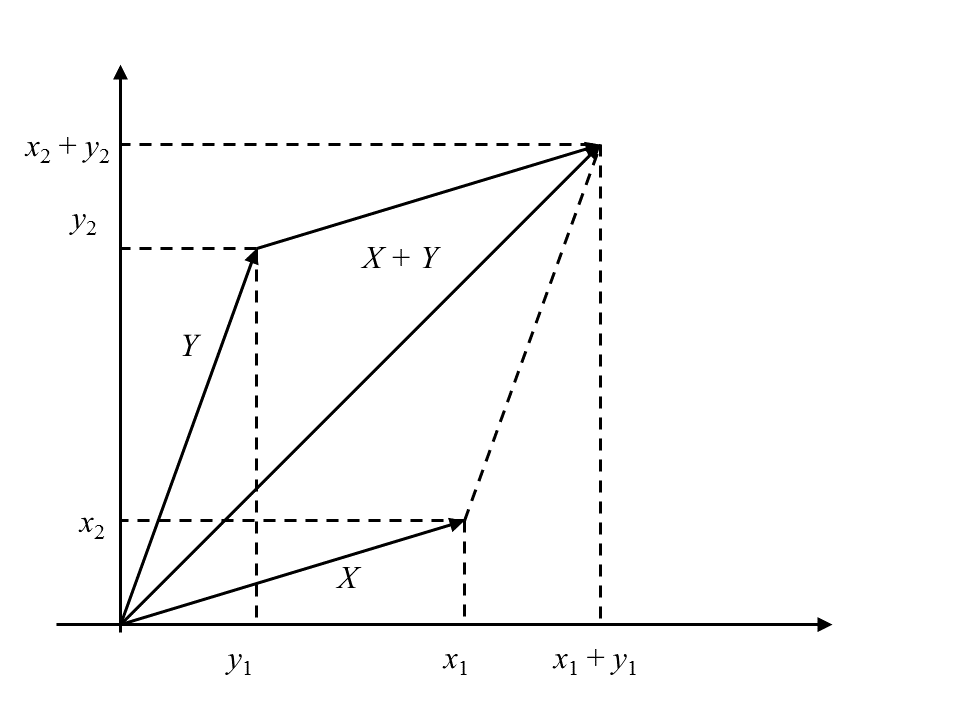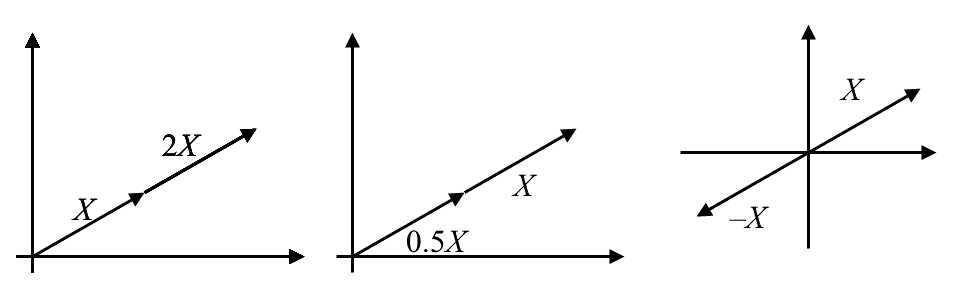7
Jul 18

## Euclidean space geometry: vector operations

The combination of these words may sound frightening. In fact, if you want to succeed with matrix algebra, you need to start drawing inspiration from geometry as early as possible.

### Sum of vectors

Definition. The set of all$n$-dimensional vectors$x=(x_1,...,x_n)$ with$x_{i}\in R$ is denoted$R^n$ and is called a Euclidean space.$R^2$ is a plane. The space we live in is$R^3.$ Our intuition doesn't work in dimensions higher than 3 but most facts we observe in real life on the plane and in the 3-dimensional space have direct analogs in higher dimensions. Keep in mind that$x=(x_1,...,x_n)$ can be called a vector or a point in$R^{n},$ depending on the context. When we think of it as a vector, we associate with it an arrow that starts at the origin$(0,...,0)$ and ends at the point$(x_1,...,x_n).$

Careful inspection shows that the sum of two vectors$x+y=(x_1+y_1,...,x_n+y_n)$ is found using the parallelogram rule in Figure 1. The rule itself comes from physics: if two forces are applied to a point, their resultant force is found by the parallelogram rule. Whatever works in real life is guaranteed to work in Math.Figure 1. Sum of vectors

Exercise 1. Let$e_1=(1,0)$ be the unit vector of the$x$ axis and$e_2=(0,1)$ the unit vector of the$y$ axis. Find the sum$e_1+e_2.$ Generalization: if$x$ runs over the whole$x$ axis and$y$ runs over the whole$y$ axis, what is the set of resulting sums$x+y?$ Further generalization: on the plane take two straight lines$L_1$ and$L_2$ that pass through the origin and are not parallel to one another. If$x$ runs over$L_1$ and$y$ runs over$L_2$, what is the set of resulting sums$x+y?$

This seemingly innocuous exercise leads to profound ideas, to be considered later. The answer for the last question is that the sums$x+y$ will cover the whole$R^{2}.$ This fact is written like this:$\{x+y:x\in L_1,\ y\in L_2\}=R^2.$ Note that I intentionally use words that emphasize movement and geometry: "runs over" and "covers the whole". One of the differences between elementary and higher mathematics is that the former deals with fixed elements and the latter with sets within which there are movement and change.

### Multiplication of a vector by a number

If$a$ is a number and$x=(x_1,...,x_n)$ is a vector, we put$ax=(ax_1,...,ax_n)$ (scaling or multiplication by a number). Scaling$x$ by a positive number$a$ means lengthening it in case$a>1$ and shortening in case$a<1$. Scaling by a negative number means, additionally, reverting the direction of$x$,  see Figure 2.Figure 2. Scaling a vector

Exercise 2. Take a nonzero vector$x=(x_1,x_2)$ and find the set of all products$ax,$ where$a$ runs over$R.$

The answer is that$\left\{ ax:a\in R\right\}$ is the straight line drawn through the vector$x$ or, alternatively, the straight line drawn through the origin and point$x.$

The expression$ax+by$ is called a linear combination of vectors$x,y$ with coefficients$a,b\in R.$ To find$ax+by,$ you first scale$x$ and$y$ and then add the results.

Exercise 3. Let$x,y$ be two nonparallel vectors on the plane. Describe the set$\left\{ ax+by:a,b\in R\right\}$ verbally and geometrically.

Verbally, this is the set of all linear combinations$ax+by$ that result when$a,b$ run over$R.$ Geometrically, this will be the whole plane$R^2.$